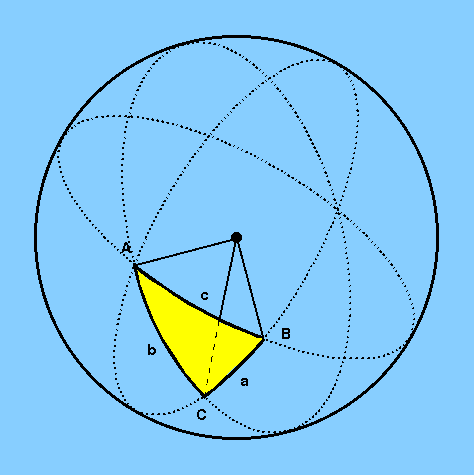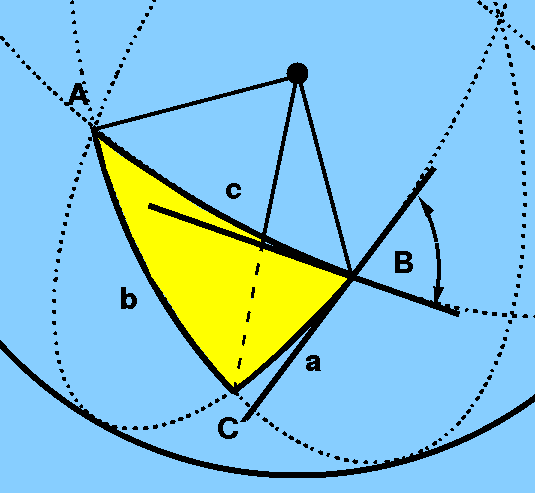To perform calculations with planar triangles it is necessary to use the formulae of planar trigonometry, which you will have met before. Similarly, to perform calculations with spherical triangles it is necessary to use the formulae of spherical trigonometry, which are given below. Figure 3 shows a spherical triangle, formed by three intersecting great circles, with arcs of length (a,b,c) and vertex angles of (A,B,C).

 figure 3: a spherical triangle with arcs of length (a,b,c) and vertex angles of (A,B,C).Note that the angle between two sides of a spherical triangle is defined as the angle between the tangents to the two great circle arcs, as shown in Figure 4 for vertex angle B.

 figure 4: the vertex angle B is defined as the angle between the tangents to the two great circle arcs.The arc lengths (a,b,c) and vertex angles (A,B,C) of the spherical triangle in Figure 4 are related by the following formulae:

The sine formula:
(sin a / sin A) = (sin b / sin B) = (sin c / sin C)

The cosine formula:
cos a = cos b cos c + sin b sin c cos A

These are the spherical analogues of the cosine and sine formulae of planar trigonometry. Their proofs are given on pages 52-54 of Roy and Clarke, along with a host of other formulae which you will not need to know - almost all of the simple problems you will encounter in spherical astronomy can be solved using just the sine and/or cosine formulae given above. The trick is to know which triangle to draw - once you have done this, the solution is easy, as shown in the example problems.